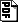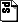An Algebraic Approach for the Unsatisfiability of Nonlinear Constraints

### Abstract

We describe a simple algebraic semi-decision procedure for detecting unsatisfiability of a (quantifier-free) conjunction of nonlinear equalities and inequalities. The procedure consists of Gr\"obner basis computation plus extension rules that introduce new definitions, and hence it can be described as a critical-pair completion-based logical procedure. This procedure is shown to be sound and refutationally complete. When projected onto the linear case, our procedure reduces to the Simplex method for solving linear constraints. If only finitely many new definitions are introduced, then the procedure is also terminating. Such terminating, but potentially incomplete, procedures are used in incompleteness-tolerant'' applications.pdf. Springer link .

#### Slides

Slides of the presentation at CSL'05 :postscriptpdf.

#### Errata

Paul Jackson correctly pointed out that Corollary 1, as stated in the paper, is wrong. The correct statement should be: P=0 AND V > 0 AND W >= 0 is unsatisfiable iff there is a polynomial p'+c\mu \in Ideal(P) \cap Cone[V,W] such that p'\in Cone[V,W] and c > 0 and \mu \in [V].

#### BibTeX Entry


@inproceedings{Tiwari05:CSL,
author = "A. Tiwari",
title = "An Algebraic Approach for the Unsatisfiability of Nonlinear Constraints",
booktitle = "Computer Science Logic, 14th Annual Conf., CSL 2005",
EDITOR = "Ong, L.",
pages = "248--262",
series = {LNCS},
volume = 3634,
PUBLISHER = {Springer},
MONTH = aug,
YEAR = 2005
}